# Swift – Set Operations

• Last Updated : 01 Feb, 2022

Set is a collection of unique values. A set can be created using the initializer or the set literal syntax. A set can also be created by adding an array of unique values to an empty set. We can use a set instead of an array when we want to remove duplicate values. A set is unordered and does not maintain indexes, so it cannot be accessed by index.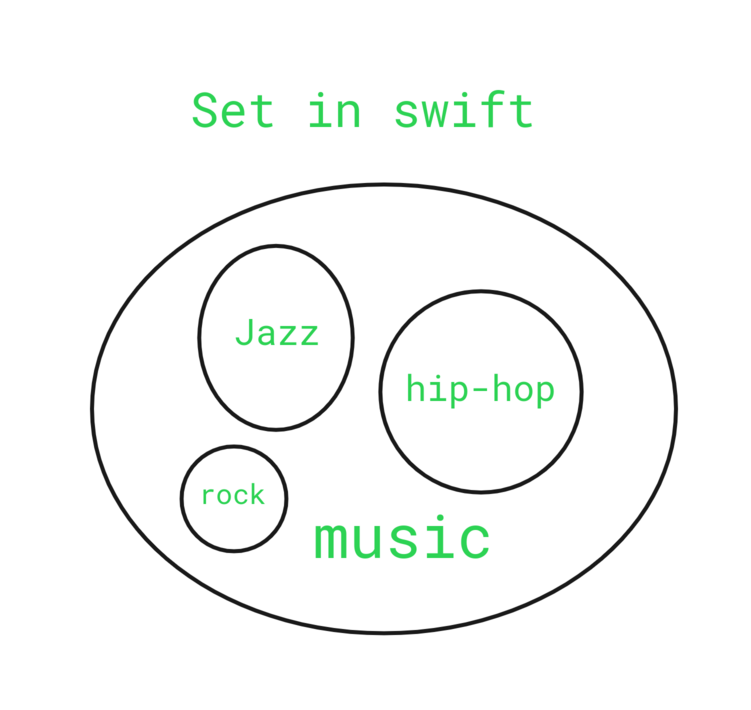Set

Here, we are using the array of music to store the music genres. The set named music contains the values rock, jazz, hip-hop, etc. In general, we can think of a set as a collection of unique values. In our example, we are taking music as an example and we are storing the values of rock, jazz, hip-hop in the set music.

Creating a Set in Swift

We can create a set with any type of values that are hashable. Hashable means that the values can be hashed and compared. In swift, strings, numeric and boolean types are hashable. To create a new set, simply use the set literal syntax.

Syntax:

var set_name: Set<type> = [value1, value2, value3, …]

Here, set_name is the name of the set and type is the type of the values in the set.

Example:

## Swift

 `// Swift program to create a set of strings``import` `Swift`` ` `// Creating a set of string``var` `setname: Set<``String``> = [ ``"a"``, ``"b"``, ``"c"``, ``"d"``, ``"e"` `] `` ` `// Displaying the set``print``(setname)`

Output:

`["a", "b", "c", "d", "e"]`

### Set Operations

In Swift, we can also perform various set operations like joining two sets together, finding the difference between two sets, checking whether a set is empty or not, and comparing two sets using their values. Or we can say Swift provides built-in methods to perform set operations like union, intersection, difference, and symmetric difference. Below is a simple diagram of various set operations.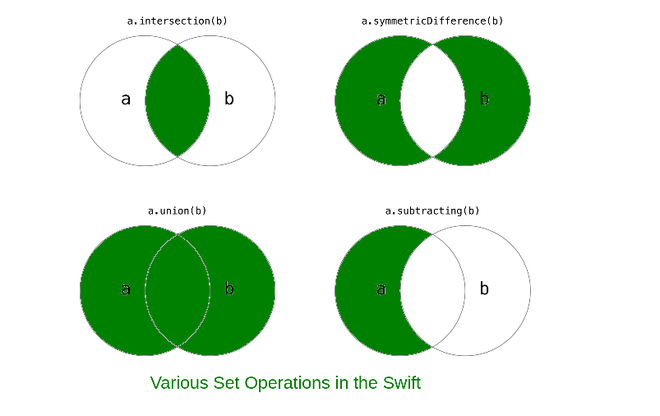### Union

Union is the set of all elements that are present in both sets, it does not contain duplicate elements. For example, we have two sets: set a and set b. So the union of both the sets contains all the elements that are in both set a and set b. Here, in the above diagram, the highlighted green portion of the figure shows that these areas are included in the resultant set. Both the set a and set b areas are included in the resultant union of the set.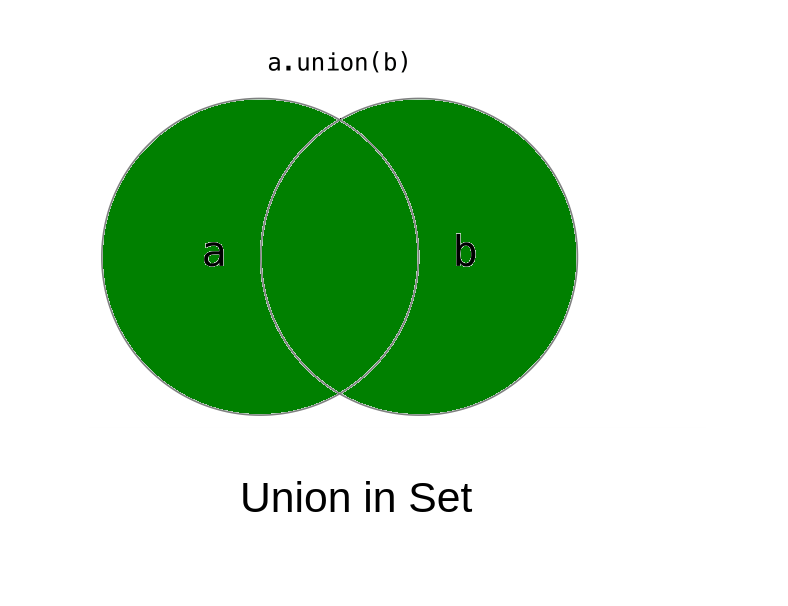Swift provides an inbuilt union() method to find the union of two sets. Or we can say that this method returns a new set that contains all the elements of set a and set b.

Syntax:

setA.union(setB)

Here, setA is the name of the first set and setB is the name of the second set.

Example:

## Swift

 `// Swift program to find the union of two sets`` ` `// Creating set A of integers``var` `setA: Set<``Int``> = [1, 2, 3, 4, 5] `` ` `// Displaying set A``print``(``"Set A:"``, setA)`` ` `// Creating set B of integers``var` `setB: Set<``Int``> = [3, 4, 5, 6, 7] `` ` `// Displaying set B``print``(``"Set B:"``, setB)`` ` `// Displaying the union of setA and setB``// Using union() method``print``(``"Union of setA and setB:"``, setA.union(setB))`

Output:

```Set A: [3, 4, 5, 2, 1]
Set B: [3, 4, 6, 5, 7]
Union of setA and setB: [4, 3, 6, 2, 5, 7, 1]```

Here, as we can see, it prints the union of the two sets i.e., [4, 3, 6, 2, 5, 7, 1]. Those elements are in either of the two sets.

### Intersection

The intersection is the set that contains only common elements present in both sets. For example, we have two sets: set a and set b. So the intersection contains the common elements present in both set a and set b. Here the highlighted green portion of the diagram shows the area that is common in both sets. So the resultant intersection of both sets has only common elements.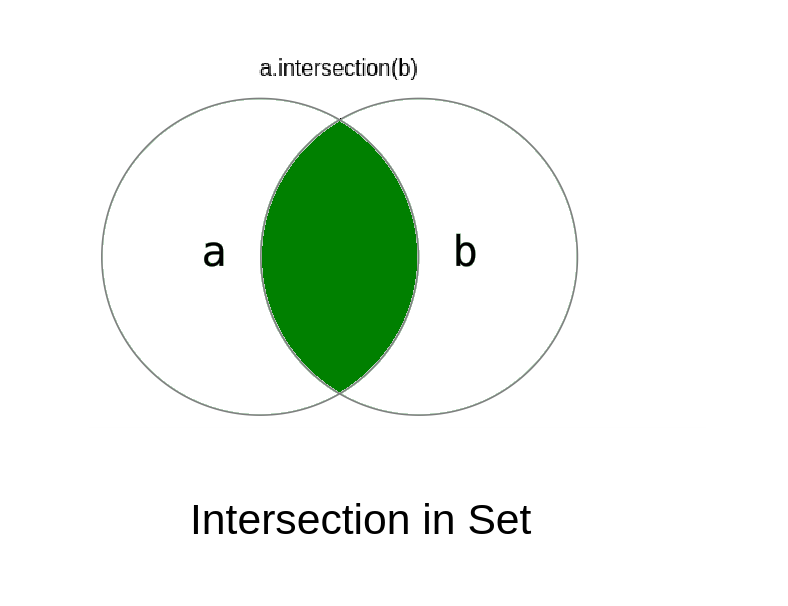Swift provides an inbuilt intersection() method to find the intersection of two sets. Or we can say that this method returns a new set that contains all the common elements of set a and set b.

Syntax:

setA.intersection(setB)

Here, setA is the name of the first set and setB is the name of the second set.

Example:

## Swift

 `// Swift program to find the intersection of two sets`` ` `// Creating set A of integers``var` `setA: Set<``Int``> = [1, 2, 3, 4, 5] `` ` `// Displaying set A``print``(``"Set A:"``, setA)`` ` `// Creating set B of integers``var` `setB: Set<``Int``> = [3, 4, 5, 6, 7] `` ` `// Displaying set B``print``(``"Set B:"``, setB)`` ` `// Displaying the intersection of setA and setB``// Using intersection() method``print``(``"Intersection of setA and setB:"``, setA.intersection(setB)) `

Output:

```Set A: [3, 2, 4, 5, 1]
Set B: [7, 3, 4, 5, 6]
Intersection of setA and setB: [4, 3, 5]```

Here, as we can see, it prints the intersection of the two sets i.e., [4, 3, 5]. Those elements are in both of the two sets only.

### Subtracting

The subtracting is the set of elements that are in the first set but not in the second set. For example, we have two sets: set a and set b. So the subtracting contains the elements present in set a but not in set b. Here, the highlighted green portion of the diagram shows the area that is included in the resultant set after the subtracting operation performed among the two sets.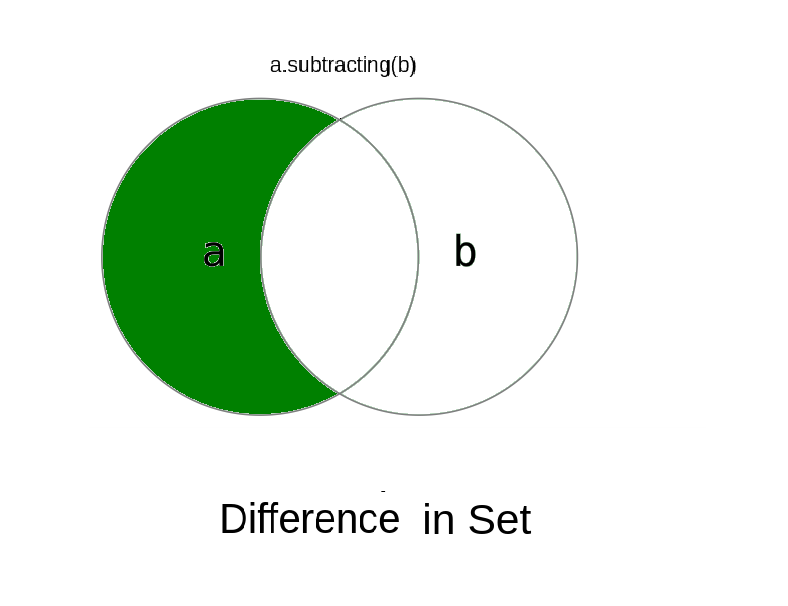Swift provides an inbuilt Subtracting() method to find the difference between two sets. Or we can say that this method returns a new set that contains the elements that are present in set a but not in set b.

Syntax:

setA.subtracting(setB)

Here, setA is the name of the first set and setB is the name of the second set.

Example:

## Swift

 `// Swift program to find the subtracting of two sets`` ` `// Creating set A of integers``var` `setA: Set<``Int``> = [1, 2, 3, 4, 5] `` ` `// Displaying set A``print``(``"Set A:"``, setA)`` ` `// Creating set B of integers``var` `setB: Set<``Int``> = [3, 4, 5, 6, 7] `` ` `// Displaying set B``print``(``"Set B:"``, setB)`` ` `// Displaying the subtracting of setA and setB``// Using subtracting() method``print``(``"Subtracting of setA and setB:"``, setA.subtracting(setB)) `

Output:

```Set A: [4, 5, 1, 2, 3]
Set B: [3, 4, 5, 7, 6]
Subtracting of setA and setB: [1, 2]```

Here, as we can see, it prints the difference of the two sets, i.e., [1, 2]. Those elements are present in the first set but not in the second set.

### Symmetric difference

The symmetric difference is the set of all elements that are in either of the two sets but not in both of them. For example, we have two sets: set a and set b. So the symmetric difference contains the elements present either of the two sets but not in both of them. Here, the highlighted green portion of the diagram shows the area that is included in the resultant set after performing the symmetric difference operation on both sets. In this operation, the elements that are common in both sets are ignored.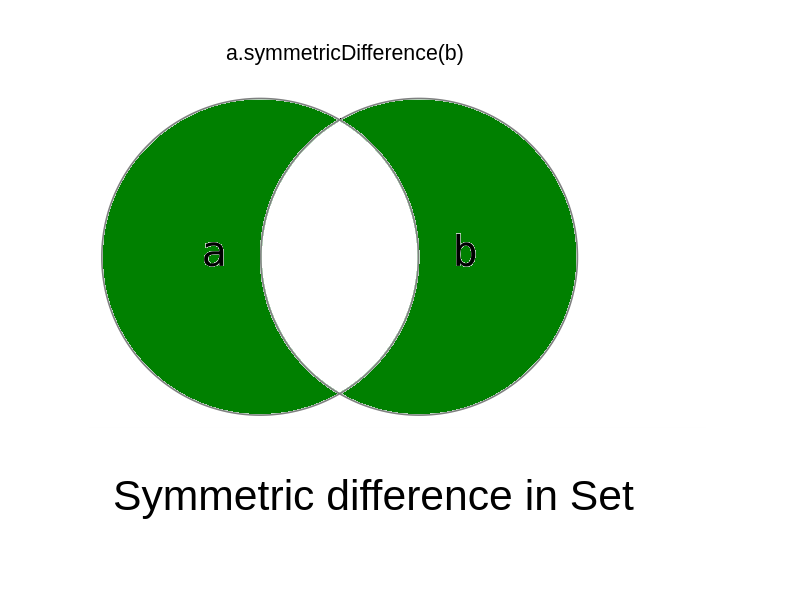Swift provides an inbuilt symmetricDifference() method to create a new set with values in either set, but not both. Where a and b represents the two set on which we are performing symmetric difference operation.

Syntax:

setA.symmetricDifference(setB)

Here, setA is the name of the first set and setB is the name of the second set.

Example:

## Swift

 `// Swift program to find the symmetricDifference of two sets`` ` `// Creating set A of integers``var` `setA: Set<``Int``> = [1, 2, 3, 4, 5] `` ` `// Displaying set A``print``(``"Set A:"``, setA)`` ` `// Creating set B of integers``var` `setB: Set<``Int``> = [3, 4, 5, 6, 7] `` ` `// Displaying set B``print``(``"Set B:"``, setB)`` ` `// Displaying the symmetricDifference of setA and setB``// Using symmetricDifference() method``print``(``"SymmetricDifference of setA and setB:"``, ``      ``setA.symmetricDifference(setB)) `

Output:

```Set A: [3, 1, 2, 4, 5]
Set B: [6, 5, 3, 7, 4]
SymmetricDifference of setA and setB: [6, 1, 2, 7]```

Here, as we can see, it prints the symmetric difference of the two sets i.e., [6, 1, 2, 7]. Those elements which are in either of the two sets but not in both of them.

### Check if two sets are equal

Swift provide an == operator. This operator is used to check if both the sets are equal or not. This operator will return true if both the sets are equal. Or it will return false if both the sets are not equal.

Syntax:

setA == setB

Here, setA is the name of the first set and setB is the name of the second set.

Example:

## Swift

 `// Swift program to check the given sets are equal or not `` ` `// Creating set A of integers``var` `setA: Set<``Int``> = [1, 2, 3, 4, 5] `` ` `// Displaying set A``print``(``"Set A:"``, setA)`` ` `// Creating set B of integers``var` `setB: Set<``Int``> = [3, 4, 5, 6, 7] `` ` `// Displaying set B``print``(``"Set B:"``, setB)`` ` `// Checking setA is equal to setB``// Using == operator ``print``(``"Is setA is equal to setB: "``, ``      ``setA == setB) `

Output:

```Set A: [2, 4, 5, 3, 1]
Set B: [4, 5, 6, 7, 3]
Is setA is equal to setB:  false```

### Check subset of a set

A set is said to be a subset of another set if all the elements of the first set are present in the second set like as shown in the below image. Swift provide a in-built method isSubset(). This method is used to check whether all the values of a set b are available in the set a. This method will return true if the set is subset otherwise it will return false.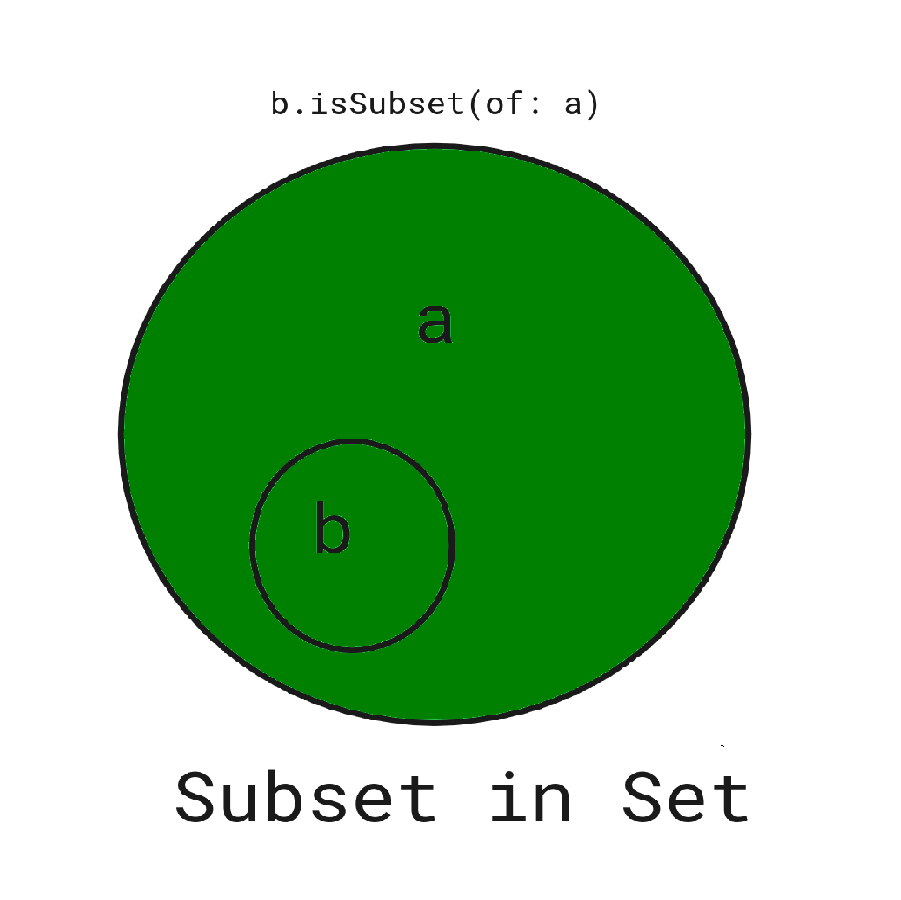Syntax:

setB.isSubset(of: setA)

Here, setB is the set which we want to check whether it is a subset of setA or not.

Example:

## Swift

 `// Swift program to check the given set is the subset or not  `` ` `// Creating set A of integers``var` `setA: Set<``Int``> = [1, 2, 3, 4, 5] `` ` `// Displaying set A``print``(``"Set A:"``, setA)`` ` `// Creating set B of integers``var` `setB: Set<``Int``> = [3, 4, 5, 6, 7] `` ` `// Displaying set B``print``(``"Set B:"``, setB)`` ` `// Checking setB is the subset of setA``// Using isSubset() method``print``(``"Is setB is the subset of setA and setB:"``, ``      ``setB.isSubset(of: setA)) `

Output:

```Set A: [3, 2, 1, 4, 5]
Set B: [4, 6, 3, 5, 7]
Is setB is the subset of setA and setB: false```

My Personal Notes arrow_drop_up